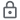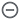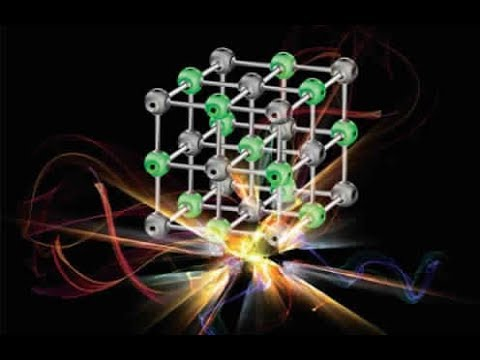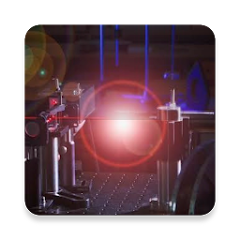# Engineering Physics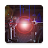10K+Everyone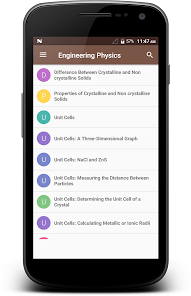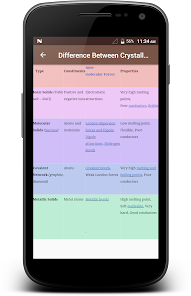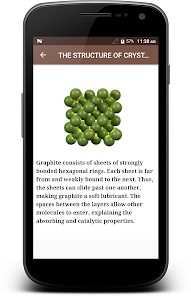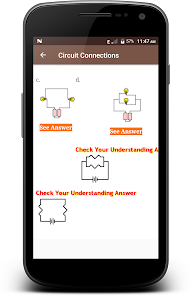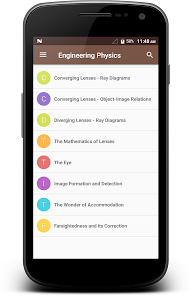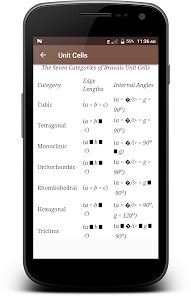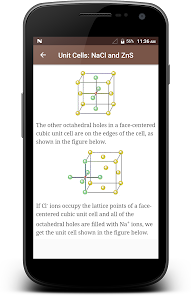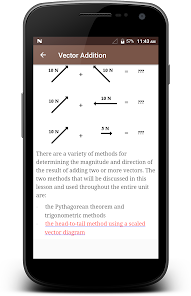✴ Engineering physics or engineering science refers to the study of the combined disciplines of physics, mathematics and engineering, particularly computer, nuclear, electrical, electronic, materials or mechanical engineering. By focusing on the scientific method as a rigorous basis, it seeks ways to apply, design, and develop new solutions in engineering.✴

►This Engineering Physics App that might be suitable for you if you are interested in newly developing areas of physics, instrumentation and communications, have an avid interest in technology and a passion for physics. Skills like problem-solving, observation skills, numerical aptitude, practical thinking and reasoning are important for this field. Technical know-how is beneficial and professionals in this field should be able to communicate their ideas to others with clarity, precision and imagination in a way that captures the interest of others. Engineering physics stresses the application of fundamental scientific principles to the design of equipment.✦

【Few Topics Covered in this App are Listed Below】

⇢ Difference Between Crystalline and Non crystalline Solids

⇢ Properties of Crystalline and Non crystalline Solids

⇢ Unit Cells: A Three-Dimensional Graph

⇢ Unit Cells: NaCl and ZnS

⇢ Unit Cells: Measuring the Distance Between Particles

⇢ Unit Cells: Determining the Unit Cell of a Crystal

⇢ Unit Cells: Calculating Metallic or Ionic Radii

⇢ THE STRUCTURE OF CRYSTALS

⇢ The Seven Crystal Systems

⇢ PROPERTIES OF MATTER AND THERMAL PHYSICS

⇢ Stress & Strain

⇢ Stress Strain Curve Explanation

⇢ Modes of Heat Transfer – Conduction, Convection & Radiation

⇢ Thermal conductivity

⇢ Newton's Law of Cooling

⇢ Kinematics

⇢ Scalars and Vectors

⇢ Distance and Displacement

⇢ Speed and Velocity

⇢ Velocity as a Vector Quantity

⇢ Calculating Average Speed and Average Velocity

⇢ Average Speed versus Instantaneous Speed

⇢ Acceleration

⇢ The Meaning of Constant Acceleration

⇢ Calculating the Average Acceleration

⇢ Free Fall Motion

⇢ Falling with Air Resistance

⇢ Double Trouble (a.k.a., Two Body Problems)

⇢ Newton's Third Law of Motion

⇢ Double Trouble in 2 Dimensions (a.k.a., Two Body Problems)

⇢ The Impulse-Momentum Change Theorem

⇢ Momentum as a Vector Quantity

⇢ Momentum Conservation Principle

⇢ Equal and Opposite Momentum Changes

⇢ Solving Explosion Momentum Problems

⇢ Analysis of Situations Involving External Forces

⇢ Analysis of Situations in Which Mechanical Energy is conserved

⇢ Application and Practice Questions

⇢ Bar Chart Illustrations

⇢ Circular Motion and Satellite Motion

⇢ Acceleration

⇢ The Centripetal Force Requirement

⇢ The Forbidden F-Word

⇢ Mathematics of Circular Motion

⇢ Grounding - the Removal of a Charge

⇢ Charge Interactions Revisited

⇢ Coulomb's Law

⇢ Inverse Square Law

⇢ Newton's Laws and the Electrical Force

⇢ Electric Fields

⇢ Electric Field Intensity

⇢ Circuit Connections

⇢ Two Types of Connections

⇢ Series Circuits

⇢ Parallel Circuits

⇢ Combination Circuits

⇢ Waves

⇢ Properties of Periodic Motion

⇢ Pendulum Motion

⇢ Motion of a Mass on a Spring

⇢ The Nature of a Wave

⇢ What is a Wave?

⇢ Categories of Waves

⇢ The Speed of a Wave

⇢ The Wave Equation

⇢ Behaviour of Waves

⇢ Reflection, Refraction, and Diffraction

⇢ Interference of Waves

⇢ The Doppler Effect

⇢ Standing Waves

⇢ Formation of Standing Waves

⇢ Nodes and Anti-nodes

⇢ Harmonics and Patterns

⇢ Mathematics of Standing Waves

⇢ Sound Waves and Music

⇢ Spherical Aberration

⇢ Convex Mirrors

⇢ Ray Diagrams - Convex Mirrors

⇢ The Direction of Bending

⇢ If I Were an Archer Fish

⇢ The Mathematics of Refraction

⇢ Snell's Law

⇢ Ray Tracing and Problem-Solving

⇢ Total Internal Reflection

⇢ The Critical Angle

⇢ Interesting Refraction Phenomena

⇢ Rainbow Formation
Updated on
Sep 29, 2022

## Data safety

Safety starts with understanding how developers collect and share your data. Data privacy and security practices may vary based on your use, region, and age. The developer provided this information and may update it over time.This app may share these data types with third parties
Device or other IDsNo data collected